# Electricity Equations

Electricity is the branch of Physics that is dedicated to the study of phenomena of an electrical nature. The equations are divided between Electrostatics and Electrodynamics.

Electrical phenomena and equipment are very present in our daily lives. Lamps , shower, air conditioning, computers, cars, among others, are some examples of equipment that works with the use of Electricity . This branch of Physics is dedicated to the study of any electrical phenomenon and is divided into Electrostatics and Electrodynamics .

See the definition of each Electricity subdivision and the main equations used:

→ Electrostatics

In this field of study, all electrical phenomena occur with electrical charges at rest.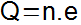Q = Electric charge (C – coulomb);

n = Number of elementary charges;

e = Elementary charge (e = 1.6 x 10 – 19 C).

• Electric force – Coulomb’s Law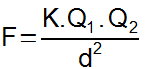F = Electric force (N – Newton);

1 and Q 2 = Electric charges (C);

d = Distance between electrical charges (m);

K = Electrostatic constant in vacuum (k = 9.0 x 10 9 Nm – 2 C – 2 ).

• Electric field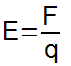E = Electric field (N/C);

F = Electric force (N);

q = Test electrical charge ©.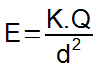E = Electric field (N/C);

K = Electrostatic constant in vacuum (k = 9.0 x 10 9 Nm – 2 C – 2 );

Q = Electric charge generating the electric field (C);

d = Distance between the electric charge and any point (m).

• electrical potential energy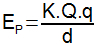P = Electric potential energy (J);

K = Electrostatic constant in vacuum (k = 9.0 x 10 9 Nm – 2 C – 2 );

Q eq = Electric charges (C);

d = Distance between electrical charges.

• Electric potential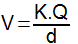V = Electric potential (V – volts);

K = Electrostatic constant in vacuum (k = 9.0 x 10 9 Nm – 2 C – 2 );

Don’t stop now… There’s more after the publicity 😉

Q = Electric charge (C);

d = Distance between the electric charge and any point (m).

→ Electrodynamics

In Electrodynamics, electrical phenomena occur with moving electrical charges. The orderly movement of electric charges is called electric current.

• Electric current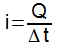i = Electric current (A = ampere);

Q = Electric charge (C);

Δ t = Time interval considered for the flow of electric charges (s).

• Ohm’s Laws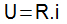U = Electric potential difference (V);

R = Electrical resistance (Ω – ohm);

i = Electric current (A).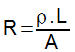R = Electrical resistance (Ω – ohm);

ρ = Resistivity (Ω .m);

L = Length of conductive material (m);

A = Cross-sectional area (m 2 ).

• Combination of resistors in series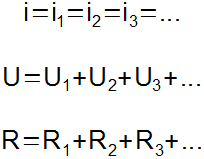i = Electric current (A);

U = Potential difference (V);

R = Electrical resistance (Ω – ohm).

• Connecting resistors in parallel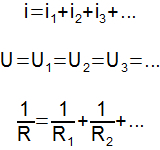i = Electric current (A);

U = Potential difference (V);

R = Electrical resistance (Ω – ohm).

• Joule effect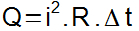Q = Amount of heat (cal – Calories);

i = Electric current (A);

R = Electrical resistance (Ω – ohm);

Δ t = Time interval (s).

• Electric power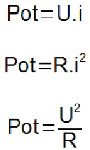Pot = Electric power (W – watts);

i = Electric current (A);

U = Potential difference (V);

R = Electrical resistance (Ω – ohm).

• Energy consumed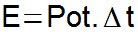E = Energy consumed (kWh – kilowatt-hour);

Pot = Power of electrical equipment (kW);

Δ t = Time interval (h).

Check Also
Close